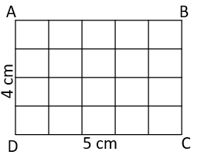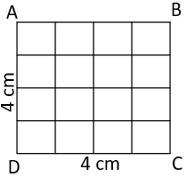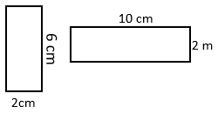## LetsPlayMaths.Com

WELCOME TO THE WORLD OF MATHEMATICS

# Class 5 Area

Area of Rectangle

Area of Square

Area Test

Area Worksheet

Answer Sheet

## Area of Rectangle

Let us have a look at the below given rectangle, whose length is 5 cm and breadth is 4 cm. Now divide the length into 5 equal parts of 1 cm each and breadth into 4 equal parts of 1 cm each.So, we find that 20 one centimeter squares completely covering the rectangle ABCD. Thus, the area of the rectangle is equal to 20 Sq. cm. In other word we can derive this as:
Area of a rectangle = Length X Breadth

Example 1. Find the area of a rectangle whose length is equal to 6.5 cm and breadth is equal to 4.5 cm.

Solution. Length of the rectangle = 6.5 cm
Breadth of the rectangle = 4.5 cm
Area of the rectangle = Length x Breadth
= 6.5 cm x 4.5 cm = 29.25 Sq. cm

## Area of Square

Let us consider below given square and find out its area.Area of a square = Side x Side = 4 cm x 4 cm = 16 Sq. cm

1 square centimeter in short is written as 1 Sq. cm or 1 cm2.
1 square meter in short is written as 1 sq. m or 1 m2.
1 square kilometer in short is written as 1 sq. km or 1 km2.
Let us have a look at some examples.

Example 1. Find the area of a square whose sides are 10 cm each.

Solution.Side of the square = 10 cm
Area of the square is = Side x Side = 10 cm x 10 cm = 100 sq. cm.
So, the area of the square is 100 sq. cm.

Example 2. Find the area of a square whose sides are 10 cm each.

Solution. Side of the square = 10 cm
Area of the square is = Side x Side = 10 cm x 10 cm = 100 sq. cm.

Example 3. Area of a square is 64 sq. cm. Find out the Side of the square.

Solution. Area of a square = Side x Side    (Side = A)

=> A x A = 64 sq. cm

=> A = 8 cm

So, one side of the square is 8 cm.

Example 4. Area of a rectangular field is 150 sq. m. Width of the rectangular field is 10 m, then find out the length of the field.

Solution. Area of a rectangle = Length x Breadth    (Length = A, Breadth = B)

=> A x B = 150

=> A x 10 = 150

=> A = 150 ũ 10

=> A = 15 m

So, length of the rectangular field is 15 m.

Example 5. The perimeter of a square is 120 cm. Find out the area of the square.

Solution. Perimeter of a square = 4 x A    (A = One side of the square)

=> 4 x A = 120

=> A = 120 ũ 4

=> A = 30 cm

One side of the square is equal to 30 cm.

Area of the square = A x A = 30 x 30 = 900 sq. cm

So, area of the square is 900 sq. cm.

Example 6. The perimeter of a rectangular garden is 54 m. If its breadth is 12 m, then find out area of the garden.

Solution. Perimeter of a rectangle = 2 x ( Length + Breadth)

Lets assume, Length = A and Breadth = B = 12 m

=> 2 x ( A + B ) = 54

=> A + B = 54 ũ 2

=> A + 12 = 27

=> A = 27  12

=> A = 15 m

Length of the field is 15 m

Area of the garden = Length x Breadth = 15 x 12

= 180 sq. m

So, area of the garden is equal to 180 sq. m.

Example 7. Find the area of the below give figure.Solution. In the above figure we can see there are two rectangular bars as shown below. One is horizontal bar and other one is vertical bar.Area of vertical bar = 6 x 2 = 12 Sq. cm

Area of the horizontal bar = 10 x 2 = 20 Sq. cm

Total area of the given figure = Area of vertical bar + Area of horizontal bar

= 12 + 20 = 32 Sq. cm

So, the area of the given figure is equal to 32 Sq. cm

Example 8. The area of a rectangular field is 700 sq. m and its length is 100 m. Find the cost of fencing it at Rs. 575 per meter.

Solution. Lets assume Length of the rectangular field is A and Breadth is B.

A = 100 m Area of the rectangular field = A x B

=> A x B = 700

=> 100 x B = 700

=> B = 700 ũ 100

=> B = 70 m

Perimeter of the rectangular field = 2 x ( A + B )

= 2 x ( 100 + 70 )

= 2 x 170

= 340 m

Total cost of fencing 340 m field = 340 x 575

= Rs. 195500

Area Test - 1

Area Test - 2

## Class-5 Area Worksheet

Area Worksheet - 1

Area Worksheet - 2

Area Worksheet - 3

Area Worksheet - 4

## Answer Sheet

Area-AnswerDownload the pdf

Copyright © 2021 LetsPlayMaths.com. All Rights Reserved.